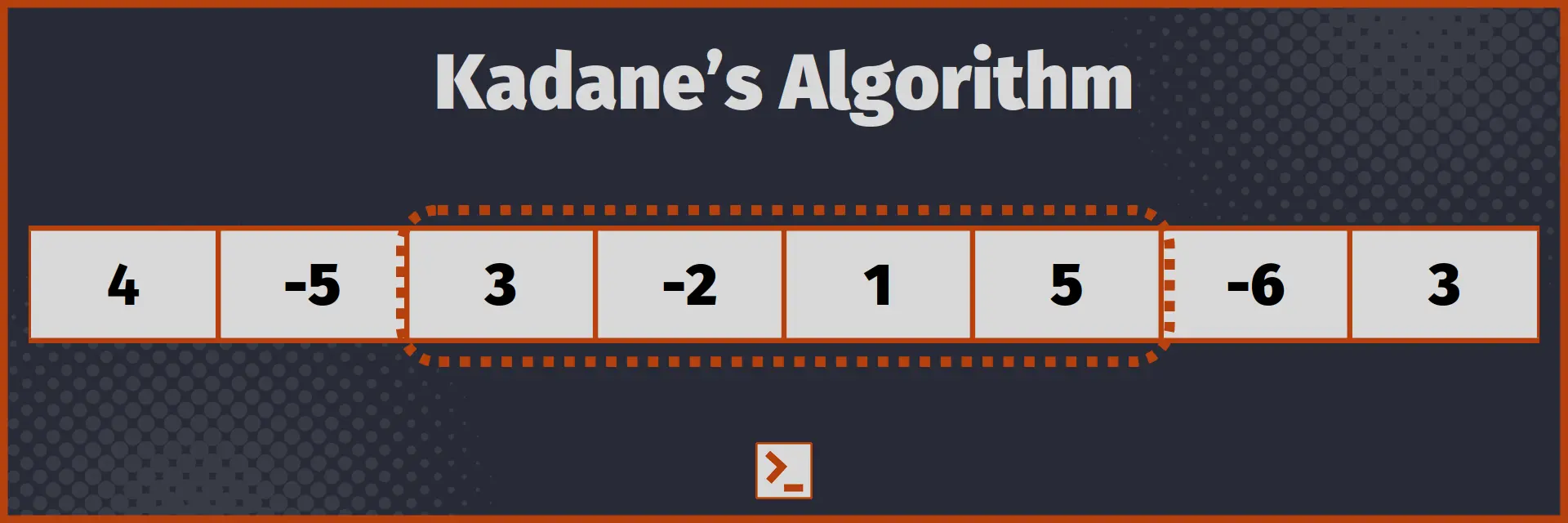# Maximum Subarray Sum

in an array using Kadane’s Algorithm in Rust

## Problem Statement

Given an array of numbers, say arr[], containing both positive and negative elements, you have to find the largest sum of the subarray of arr[].

Subarray is defined as contiguous part of the original array, containing one or more element. For example, for array [1, 2, 3], [1, 2] is subarray while [1, 3] is not.

We have to find the largest sum of all the subarray present in the given arr[].## Naive Approach

Naive or brute force approach is to find the sum of all the subarray and return the maximum of them. Function using this approach is

```fn max_subarray_sum(arr : Vec<i128>) -> i128{
// it is not initialized to 0 because array can be all negative elements.    let mut max_sum = arr;
// Traverse through all the subarray    for i in 0..arr.len() {
// We use from i+1 to N inclusive        // Because j is ending range of the slice.        for j in i+1..arr.len()+1 {
// We find the sum of the subarray [i..j]            let mut sum = 0;            for k in i..j {                sum+=arr[k];            }
// Now compare the sum of this subarray with the max_sum            if max_sum < sum {                max_sum = sum;            }        }    }
return max_sum;}```

With driver code

```fn max_subarray_sum(arr : Vec<i128>) -> i128{
// it is not initialized to 0 because array can be all negative elements.    let mut max_sum = arr;
// Traverse through all the subarray    for i in 0..arr.len() {
// We use from i+1 to N inclusive        // Because j is ending range of the slice.        for j in i+1..arr.len()+1 {
// We find the sum of the subarray [i..j]            let mut sum = 0;            for k in i..j {                sum+=arr[k];            }
// Now compare the sum of this subarray with the max_sum            if max_sum < sum {                max_sum = sum;            }        }    }
return max_sum;}
// Driver Code
fn main() {    let arr:Vec<i128> = vec![4, -5, 3, -2, 1, 5, -6, 3];    println!("{}", max_subarray_sum(arr));}```

Output

7

Time Complexity : O( n3 )
Space Complexity : O( 1 )

Using Kadane’s Algorithm, we can find Maximum Subarray Sum in Linear time complexity and constant space complexity.

### Observation

In Kadane’s Algorithm, we use a simple Observation, that if the sum of the elements upto a given element is negative, we can discard this sum. For example, in array,

[4, -5, 3, -2, 1, 5, -6, 3]

We can see that sum of subarray [4, -5] is negative. So, we can easily discard this, because it will only decrease the sum of the elements. We can not include 4 without including -5. So, it is better to drop this subarray.

But in the array

[4, -3, 3, -2, 1, 5, -6, 3]

We can include the subarray [4, -3] because its sum is positive.

### Algorithm

1. Initialise max_sum to any element of arr[] and current_sum to 0.
2. Add the first element to the current_sum.
3. If current_sum is greater than the max_sum, set max_sum to current sum.
4. If current sum is less than 0, set current_sum = 0.
5. Repeat step 2 to 5 for each element of the arr[] and return the max_sum.

Function using this approach is

```fn max_subarray_sum(arr : Vec<i128>) -> i128{
// max sum is not initialized to 0 because array can be all negative elements.    let mut max_sum = arr;    let mut current_sum = 0;

for i in 0..arr.len() {        // We add element to the current sum first        // because we have to consider single element also        current_sum+=arr[i];
// If current sum is greater than max sum, it becomes max.        if current_sum>max_sum {            max_sum = current_sum;        }
// Discard the current sum if it is less than 0        if current_sum < 0 {            current_sum = 0;        }    }
return max_sum;}```

Use the same driver code.

Output

7

Time Complexity : O( n )
Space Complexity : O( 1 )

## Conclusion

Kadane's Algorithm is used to find the Maximum Subarray Sum in an array that may have positive as well as negative integers.

In this article, we saw the Kadane's Algorithm and also wrote the function to find the Maximum Subarray Sum in Rust Language.

Here is the optimized function for easy access

`fn max_subarray_sum(arr : Vec<i128>) -> i128{    let mut mx = arr;    let mut curr = 0;    for i in 0..arr.len() {        curr+=arr[i];        if curr>mx { mx = curr; }        if curr < 0 { curr = 0; }    }    return mx;}`

Thank You